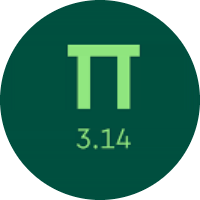# Understanding the Monomials and Polynomials

In this quiz, you will get to know about the monomials and polynomials. You will learn about identifying the monomials, adding, subtracting, multiplying, and dividing the monomials. You also learn about multiplying polynomials.

Start Quiz

Is r + 5s − 4 − 2t a monomial?

yes

no

Subtract.

(7q² + 9q + 5) - (q² + 4q + 3)

6q² + 5q + 2

4q² + 6q + 2

6q² + 4q + 7

3q² + 5q + 5

(7q + 5) + (5q + 8)

14q + 8

12q + 13

13q + 12

8q + 13

Simplify.

(2p).(6p⁴)

12p⁴

16p⁶

12p⁵

16p⁴

Simplify.

4sp⁷ / 8sp⁵

p²/2

p⁵/5

p²/4

Simplify:

(6s⁵)²

36s²⁵

6s¹⁰

36s¹⁰

Evaluate.

∛(64s²⁷)

4s³

4s⁹

8s⁸

-3y(-3y² - 2)

9y³ + 6y

9y² + 6y

9y + 6

Find the product.

(2p + 4).(2p - 4)

4p² - 10

4p - 16

4p² - 16

Quiz/Test Summary
Title: Understanding the Monomials and Polynomials
Questions: 9
Contributed by: